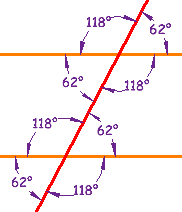1. what is equiform geometry?2.

3. Google the term and you will get many hits. In one of them it seems to be what is taught as high school geometry.I know that it's basic geometry of attributes of geometric bodies that doesn't change by the same movements.
what the means of "movements"?
is it the meaning of transofrmation?!
What the difference between basic geometry to equiform geometry?
is it a part of basic geometry?!

Thanks.5.Originally Posted by Shaharhada
I know that it's basic geometry of attributes of geometric bodies that doesn't change by the same movements.
what the means of "movements"?
is it the meaning of transofrmation?!
What the difference between basic geometry to equiform geometry?
is it a part of basic geometry?!

Thanks.
It's basic geometry, I suggest you take an introductory class in geometry if you don't understand, it'll be much more helpful than we will on this forum. The one-on-one time with an instructor will help you.6. I not lived in a place that have the information about equiform geometry.
I wait for info about it.
I have only Hebrew book in the labrory and I ask in Hebrew forums but they don't know and they are learning in the university. (So if they didn't know who know)7.Originally Posted by Shaharhada
I not lived in a place that have the information about equiform geometry.
I wait for info about it.
I have only Hebrew book in the labrory and I ask in Hebrew forums but they don't know and they are learning in the university. (So if they didn't know who know)
I'll repeat what I said before. You will get a lot more information and in depth by using Google or Bing to search the internet.8. From where I will start?!9. it's basic geometry. If your hebrew universities have geometry, then you will learn. Every link I've seen shows equiform geometry to be roughly equivalent to euclidean geometry. It appears to have originated in the far-east (Japan, China). Try looking there.10.Originally Posted by Shaharhada
what is equiform geometry?
Shaharhada , just an educated guess here:

equi- = equal, same

-form = structure, configuration

Thus,

equiform = similar shapes

So, geometry teachers and books will state things such as:

A straight line intersecting two parallel straight lines will create identical angles with them.The equiform idea here is that the angles created in this way would be "identical".

Triangles with at least two identical angles and at least one identical side, or at least one identical angle and two identical sides, are congruent.The equiform idea here is "congruency" (ie, identical in every way - angles, sides, perimeter, area, etc).